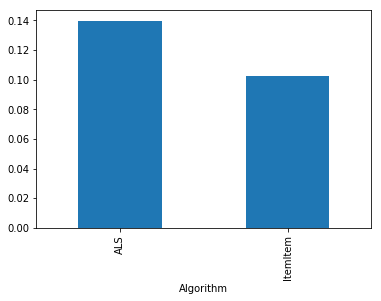# Getting Started¶

This notebook gets you started with a brief nDCG evaluation with LensKit for Python.

This notebook is also available on Google Collaboratory and nbviewer.

## Setup¶

We first import the LensKit components we need:

In :
from lenskit.datasets import ML100K
from lenskit import batch, topn, util
from lenskit import crossfold as xf
from lenskit.algorithms import Recommender, als, item_knn as knn
from lenskit import topn


And Pandas is very useful:

In :
import pandas as pd

In :
%matplotlib inline


We're going to use the ML-100K data set:

In :
ml100k = ML100K('ml-100k')
ratings = ml100k.ratings

Out:
user item rating timestamp
0 196 242 3 881250949
1 186 302 3 891717742
2 22 377 1 878887116
3 244 51 2 880606923
4 166 346 1 886397596

## Defining Algorithms¶

Let's set up two algorithms:

In :
algo_ii = knn.ItemItem(20)
algo_als = als.BiasedMF(50)


## Running the Evaluation¶

In LensKit, our evaluation proceeds in 2 steps:

1. Generate recommendations
2. Measure them

If memory is a concern, we can measure while generating, but we will not do that for now.

We will first define a function to generate recommendations from one algorithm over a single partition of the data set. It will take an algorithm, a train set, and a test set, and return the recommendations.

Note: before fitting the algorithm, we clone it. Some algorithms misbehave when fit multiple times.

Note 2: our algorithms do not necessarily implement the Recommender interface, so we adapt them. This fills in a default candidate selector.

The code function looks like this:

In :
def eval(aname, algo, train, test):
fittable = util.clone(algo)
fittable.fit(train)
users = test.user.unique()
# now we run the recommender
recs = batch.recommend(fittable, users, 100)
# add the algorithm name for analyzability
recs['Algorithm'] = aname
return recs


Now, we will loop over the data and the algorithms, and generate recommendations:

In :
all_recs = []
test_data = []
for train, test in xf.partition_users(ratings[['user', 'item', 'rating']], 5, xf.SampleFrac(0.2)):
test_data.append(test)
all_recs.append(eval('ItemItem', algo_ii, train, test))
all_recs.append(eval('ALS', algo_als, train, test))


With the results in place, we can concatenate them into a single data frame:

In :
all_recs = pd.concat(all_recs, ignore_index=True)

Out:
item score user rank Algorithm
0 285 4.543364 5 1 ItemItem
1 1449 4.532999 5 2 ItemItem
2 1251 4.494639 5 3 ItemItem
3 114 4.479512 5 4 ItemItem
4 166 4.399639 5 5 ItemItem

To compute our analysis, we also need to concatenate the test data into a single frame:

In :
test_data = pd.concat(test_data, ignore_index=True)


We analyze our recommendation lists with a RecListAnalysis. It takes care of the hard work of making sure that the truth data (our test data) and the recoommendations line up properly.

We do assume here that each user only appears once per algorithm. Since our crossfold method partitions users, this is fine.

In :
rla = topn.RecListAnalysis()
results = rla.compute(all_recs, test_data)

/home/MICHAELEKSTRAND/anaconda3/envs/lkpy-dev/lib/python3.7/site-packages/pandas/core/indexing.py:1494: PerformanceWarning: indexing past lexsort depth may impact performance.
return self._getitem_tuple(key)

Out:
ndcg
user Algorithm
1 ALS 0.265268
ItemItem 0.259708
2 ALS 0.148335
ItemItem 0.081890
3 ALS 0.026615

Now we have nDCG values!

In :
results.groupby('Algorithm').ndcg.mean()

Out:
Algorithm
ALS         0.139689
ItemItem    0.102075
Name: ndcg, dtype: float64
In :
results.groupby('Algorithm').ndcg.mean().plot.bar()

Out:
<matplotlib.axes._subplots.AxesSubplot at 0x7f03842f8860>In [ ]: